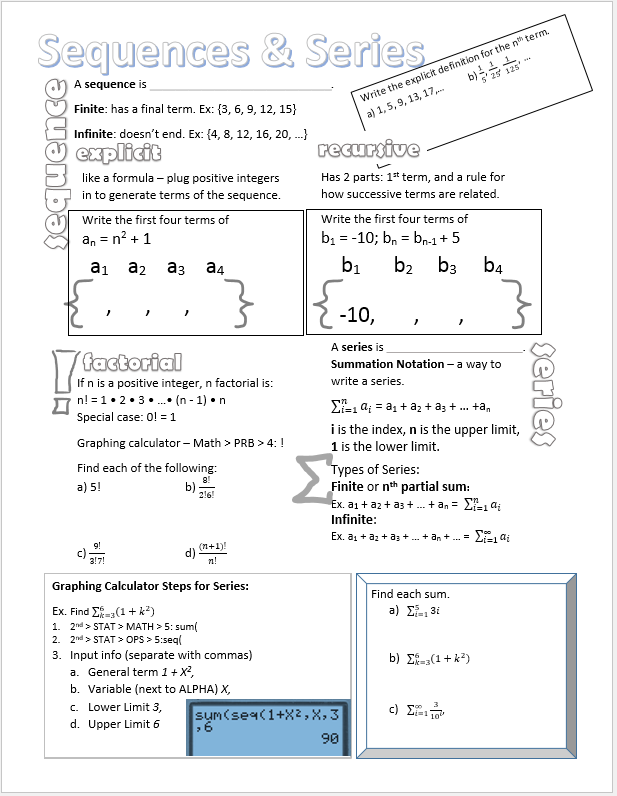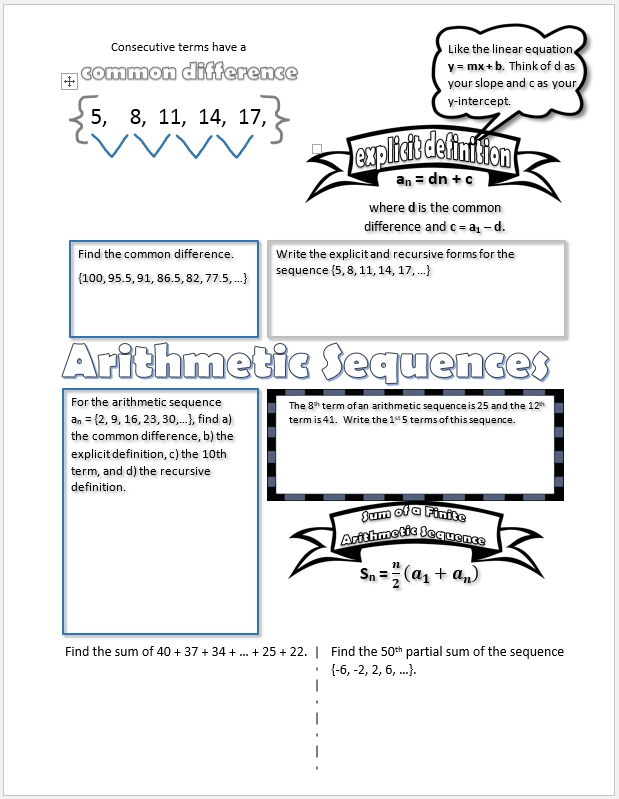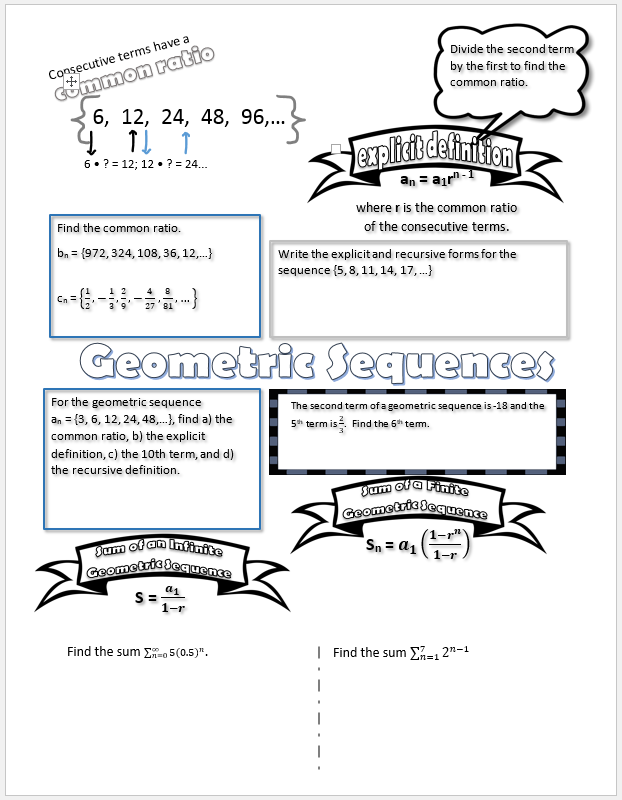# Sequences, Series, & Probability

/, PreCalculus/Sequences, Series, & Probability

## Sequences, Series, & Probability

Based on feedback from my geometry students, I’ve created some Vizual Notes for my pre-calculus classes as well.

# Sequences and Series

This notes sheet can be used to help explain the the vocabulary involved withe sequences and series – explicit, recursive, summation, factorial, etc. It helps prepare students for Arithmetic and Geometric Sequences.# Arithmetic Sequences

This Vizual Notes sheet takes students through finding common differences, writing the explicit and recursive forms for a given sequence, and finite and partial sums.# Geometric Sequences

I’ll be using this notes sheet to explain geometric sequences by finding a common ratio, the explicit and recursive forms, and using the sums of infinite and finite geometric sequences formulas.By | 2019-02-07T20:55:56+00:00 November 13th, 2016|Algebra II, PreCalculus|0 Comments

### About the Author: Stacie Bender